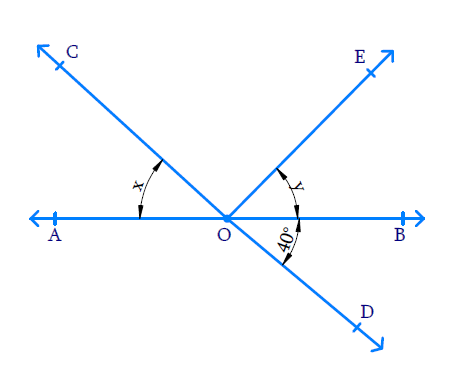# Ex.6.1 Q1 Lines and Angles Solution - NCERT Maths Class 9

## Question

In the given figure lines $$AB$$ and $$CD$$ intersect at $$O.$$ If  \begin{align} \angle AOC + \angle BOE = 70 ^ { \circ } \end{align} and \begin{align} \angle BOD = 40 ^ { \circ }, \end{align} find $$\angle BOE$$ and reflex $$\angle COE$$.Video Solution
Lines And Angles
Ex 6.1 | Question 1

## Text Solution

What is known?

$$\angle AOC+\angle BOE={70}^{\circ}$$ and $$\angle BOD = 40^{\circ}$$

What is unknown?

$$\angle BOE =?,$$ Reflex $$\angle COE =?$$

Reasoning:

We know that vertically opposite angles formed when two lines intersect are equal. Also, sum of the adjacent angles is $$180$$ degrees.

Steps:

Let $$\Delta AOC = \rm x$$ and $$\rm{}\Delta BOE =\rm y.$$

Then $$x+ y=70^\circ$$ $$(\because AOC+BOE=70^\circ )$$

Let Reflex $$\Delta COE = \rm z$$

We can see that $$AB$$ and $$CD$$ are two intersecting lines so the pair of angles formed are vertically opposite angles and they are equal.

$$\text{i.e. } \angle AOD$$ and $$\angle BOC$$ and $$\angle AOC = \angle BOD.$$

Since $$\angle AOC = x$$  and $$\angle AOC = \angle BOD = 40^ {\circ},$$

we can say that  $$x = 40^ {\circ}.$$

Also we know that,

\begin{align} x + y &= 70 ^ {\circ } \\ 40 ^ { \circ } + y &= 70 ^ {\circ } \\ y &= 70 ^ { \circ } - 40 ^ {\circ } \\ y &= 30 ^ { \circ } \\ \angle BOE &= 30 ^ { \circ } \end{align}

If we consider line $$AB$$ and ray $$OD$$ on it, then $$\angle AOD$$ and $$\angle BOD$$ are adjacent angles.

\begin{align} \angle AOD + \angle BOD &= 180 ^ { \circ } \\ \angle AOD + 40 ^ { \circ } &= 180 ^ { \circ } \\ \angle AOD &= 180 ^ { \circ } - 40 ^ { \circ } \\ &= 140 ^ { \circ } \end{align}

\begin{align}\text{Reflex }\angle COE &=\begin{pmatrix}\angle AOC +\\ \angle AOD +\\ \angle BOD +\\ \angle BOE \end{pmatrix} \\ &= \begin{pmatrix}40 ^ { \circ } + 140 ^ { \circ } \\+ 40 ^ { \circ } + 30 ^ { \circ } \end{pmatrix}\\ &= 250 ^ { \circ } \end{align}

Video Solution
Lines And Angles
Ex 6.1 | Question 1
Learn from the best math teachers and top your exams

• Live one on one classroom and doubt clearing
• Practice worksheets in and after class for conceptual clarity
• Personalized curriculum to keep up with school